## Remove text before or after first or last specific character from text strings

This tutorial will talk about how to remove the text before or after the first or last specific character, such as space, comma from a list of text strings in Excel.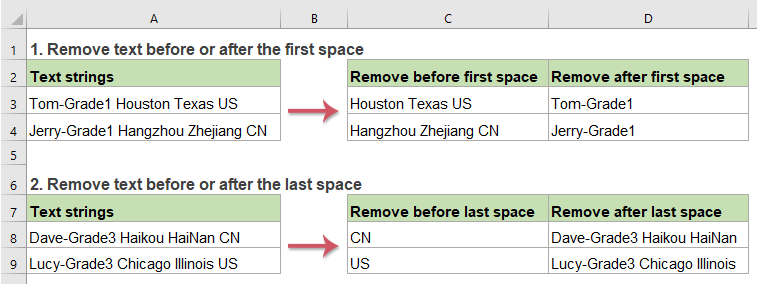#### Remove text before or after the first specific character from text strings

##### Remove text before the first specific character (such as space, comma)

To remove the text before the first specific character from text strings, the RIGHT, LEN and FIND functions can help you, the generic syntax is:

=RIGHT(cell, LEN(cell)-FIND("char", cell))
• cell: The cell reference or text string that you want to remove text from.
• char: The specific separator that you want to remove text based on.

Please enter or copy the below formula into a blank cell where you want to output the result:

=RIGHT(A2,LEN(A2)-FIND(" ",A2))

Then, drag the fill handle down to the cells that you want to apply this formula, and all the texts before the first space have been removed from the text strings, see screenshot: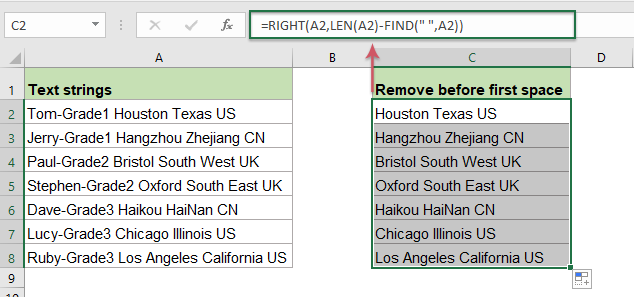##### Explanation of the formula:

1.LEN(A2)-FIND(" ",A2): This part of the formula is recognized as the num_chars within the RIGHT function.

• FIND(" ",A2): The FIND function is used to get the position of the first space, it returns the number 11.
• LEN(A2): This LEN function will return the total number of the characters in cell A2. It will get the number 27.
• LEN(A2)-FIND(" ",A2)=27-11: Subtract the position of the first space from the total length of cell A2 will get the number of the characters which after the first space.

2. RIGHT(A2,LEN(A2)-FIND(" ",A2))=RIGHT(A2, 16): Finally, this RIGHT function will extract 16 characters from right side of the text string in cell A2.

##### Remove text after the first specific character (such as space, comma)

In Excel, with the combination of the LEFT and FIND functions, you can quickly remove the text after the first specific character. The generic syntax is:

=LEFT(cell,FIND("char",cell)-1)
• cell: The cell reference or text string that you want to remove text from.
• char: The specific separator that you want to remove text based on.

Please apply the following formula into a blank cell:

=LEFT(A2,FIND(" ",A2)-1)

And then, drag the fill handle down to the cells to apply this formula, and all the texts after the first space have been removed at once, see screenshot: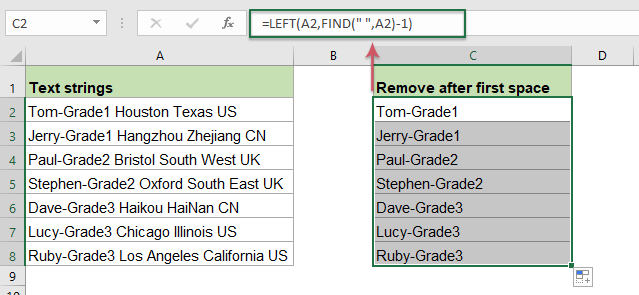##### Explanation of the formula:

1.FIND(" ",A2)-1: This FIND function will get the position of the first space in cell A2, subtracting 1 means to exclude the space character. It will get the result 10. It is recognized as the num_chars within the LEFT function.

2. LEFT(A2,FIND(" ",A2)-1)=LEFT(A2, 10): This LEFT function will extract 10 characters from left side of the text string in cell A2.

#### Remove text before or after the last specific character from text strings

##### Remove text before the last specific character (such as space, comma)

If you need to remove all the texts before the last specific character, and only leave the substrings after the last specific character, the RIGHT, LEN, SEARCH and SUSTITUTE function may do you a favor. The generic syntax is:

=RIGHT(cell,LEN(cell)-SEARCH("#",SUBSTITUTE(cell,"char","#",LEN(cell)-LEN(SUBSTITUTE(cell,"char","")))))
• cell: The cell reference or text string that you want to remove text from.
• char: The specific separator that you want to remove text based on.

To solve this task, please copy or type the following formula into a blank cell:

=RIGHT(A2,LEN(A2)-SEARCH("#",SUBSTITUTE(A2," ","#",LEN(A2)-LEN(SUBSTITUTE(A2," ","")))))

And then, drag the fill handle to fill this formula to other cells you need, and now, all texts before the last space have been deleted, only the texts after the last space kept, see screenshot: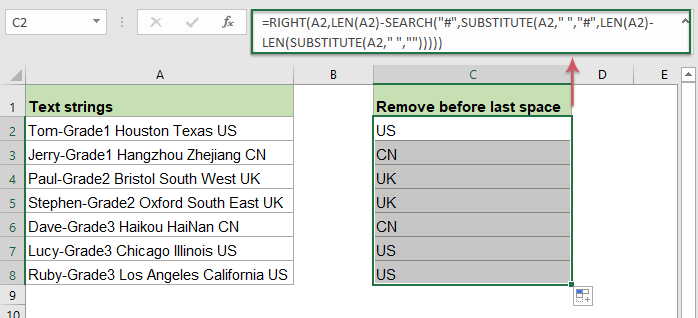##### Explanation of the formula:

1. LEN(A2)-SEARCH("#",SUBSTITUTE(A2," ","#",LEN(A2)-LEN(SUBSTITUTE(A2," ","")))):

• SUBSTITUTE(A2," ",""): This SUBSTITUTE function is used to replace all space characters with nothing in cell A2, and you will get the result as this: “Tom-Grade1HoustonTexasUS”.
• LEN(SUBSTITUTE(A2," ",""): This LEN function returns the number of characters of the text string that returned by the SUBSTITUTE function without spaces. It will get the number 24.
• LEN(A2)-LEN(SUBSTITUTE(A2," ","")=27-24: Subtract the number of characters without space from the total length of cell A2 will get the number of the spaces between the text string. This will get the number 3.
• SUBSTITUTE(A2," ","#",LEN(A2)-LEN(SUBSTITUTE(A2," ",""))= SUBSTITUTE(A2," ","#", 3): This SUBSTITUTE function is used to replace the last space character with a specific # character, the result is: “Tom-Grade1 Houston Texas#US”.
• SEARCH("#",SUBSTITUTE(A2," ","#",LEN(A2)-LEN(SUBSTITUTE(A2," ",""))))= SEARCH("#", "Tom-Grade1 Houston Texas#US") : This SEARCH function will find the position of the specific # character within the text string returned by the SUBSTITUTE function. It will get the number 25.
• LEN(A2)-SEARCH("#",SUBSTITUTE(A2," ","#",LEN(A2)-LEN(SUBSTITUTE(A2," ",""))))=27-25: The total length of the text in cell A2 subtracts the position of last space, it will get the number of the characters which after the last space. And this will get the number 2.

2. RIGHT(A2,LEN(A2)-SEARCH("#",SUBSTITUTE(A2," ","#",LEN(A2)-LEN(SUBSTITUTE(A2," ","")))))=RIGHT(A2, 2): Finally, this RIGHT function will extract 2 characters from right side of the text string in cell A2.

##### Remove text after the last specific character (such as space, comma)

To remove the text after the last specific character, the LEFT, FIND, SUBSTITUTE and LEN functions can help you, the generic syntax is:

=LEFT(cell,FIND("#",SUBSTITUTE(cell,"char","#",LEN(cell)-LEN(SUBSTITUTE(cell,"char",""))))-1)
• cell: The cell reference or text string that you want to remove text from.
• char: The specific separator that you want to remove text based on.

Please enter or copy the following formula into a blank cell:

=LEFT(A2,FIND("#",SUBSTITUTE(A2," ","#",LEN(A2)-LEN(SUBSTITUTE(A2," ",""))))-1)

And then, drag the fill handle down to the cells you want to apply this formula, and you will get the result as below screenshot shown: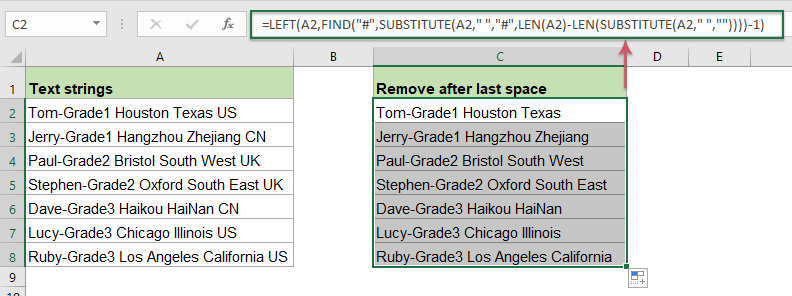##### Explanation of the formula:

1. FIND("#",SUBSTITUTE(A2," ","#",LEN(A2)-LEN(SUBSTITUTE(A2," ",""))))-1:

• SUBSTITUTE(A2," ",""): This SUBSTITUTE function is used to replace all space characters with nothing in cell A2, and you will get the result as this: “Tom-Grade1HoustonTexasUS”.
• LEN(SUBSTITUTE(A2," ",""): This LEN function returns the number of characters of the text string that returned by the SUBSTITUTE function without spaces. It will get the number 24.
• LEN(A2)-LEN(SUBSTITUTE(A2," ",""))=27-24: Subtract the number of characters without space from the total length of cell A2 will get the number of the spaces between the text string. This will get the number 3.
• SUBSTITUTE(A2," ","#",LEN(A2)-LEN(SUBSTITUTE(A2," ","")))= SUBSTITUTE(A2," ","#", 3): This SUBSTITUTE function is used to replace the last space character with a specific # character, the result is: “Tom-Grade1 Houston Texas#US”.
• FIND("#",SUBSTITUTE(A2," ","#",LEN(A2)-LEN(SUBSTITUTE(A2," ",""))))-1=FIND("#", "Tom-Grade1 Houston Texas#US ")-1=25-1: This FIND function will return the position of the specific # character in the text string which returned by the SUBSTITUTE function, subtracting 1 means to exclude the space character. And this will get the number 24.

2. LEFT(A2,FIND("#",SUBSTITUTE(A2," ","#",LEN(A2)-LEN(SUBSTITUTE(A2," ",""))))-1)=LEFT(A2, 24): At last, this LEFT function is used to extract 24 characters from left side of the text string of cell A2.

#### Relative functions used:

• LEFT:
• The LEFT function extracts the given number of characters from the left side of a supplied string.
• RIGHT:
• The RIGHT function is used to extract a specific number of characters from the right side of the text string.
• FIND:
• The FIND function is used to find a string within another string, and returns the starting position of the string inside another one.
• SEARCH:
• The SEARCH function can help you to find the position of a specific character or substring from the given text string.
• SUBSTITUTE:
• The Excel SUBSTITUTE function replaces text or characters within a text string with another text or characters.
• LEN:
• The LEN function returns the number of characters in a text string.

### The Best Office Productivity Tools

#### Kutools for Excel - Helps You To Stand Out From Crowd

Would you like to complete your daily work quickly and perfectly? Kutools for Excel brings 300 powerful advanced features (Combine workbooks, sum by color, split cell contents, convert date, and so on...) and save 80% time for you.

• Designed for 1500 work scenarios, helps you solve 80% Excel problems.
• Reduce thousands of keyboard and mouse clicks every day, relieve your tired eyes and hands.
• Become an Excel expert in 3 minutes. No longer need to remember any painful formulas and VBA codes.
• 30-day unlimited free trial. 60-day money back guarantee. Free upgrade and support for 2 years.#### Office Tab - Enable Tabbed Reading and Editing in Microsoft Office (include Excel)

• One second to switch between dozens of open documents!
• Reduce hundreds of mouse clicks for you every day, say goodbye to mouse hand.
• Increases your productivity by 50% when viewing and editing multiple documents.
• Brings Efficient Tabs to Office (include Excel), Just Like Chrome, Firefox, And New Internet Explorer.